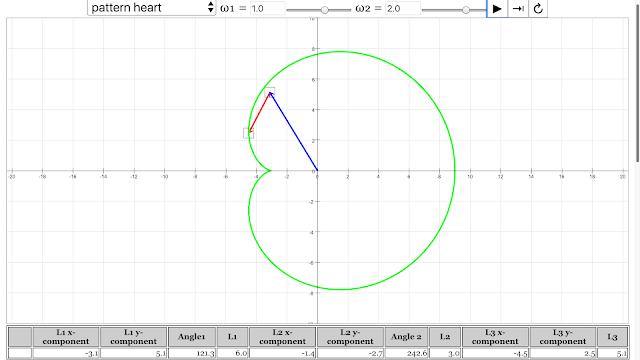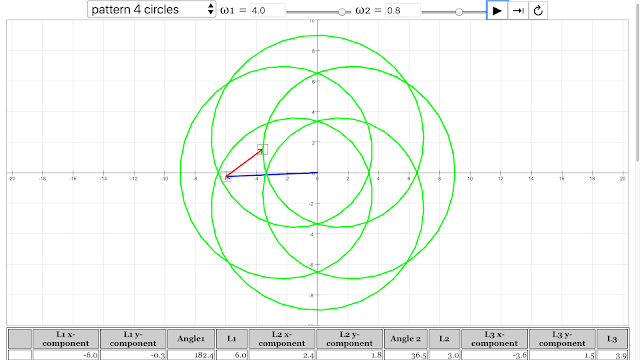{source}

<?phprequire_once JPATH_SITE.'/TTcustom/TT_contentparser.php';$$parameters = array("topicname" => "01_measurement","modelname" => "ejss_model_vector_addition_sim2_v4");echo generateSimHTML($$parameters, "EJSS");
?>
{/source}

### AAAS Benchmark Alignments (2008 Version)

#### 11. Common Themes

11B. Models
• 9-12: 11B/H2. Computers have greatly improved the power and use of mathematical models by performing computations that are very long, very complicated, or repetitive. Therefore, computers can reveal the consequences of applying complex rules or of changing the rules. The graphic capabilities of computers make them useful in the design and simulated testing of devices and structures and in the simulation of complicated processes.

### For TeachersThe pattern of coil emerged from angular velocity of vector 1 = 0.3 rad/s, angular velocity of vector 2 = 6.3 rad/s Vector Addition Pattern Creativity Lesson JavaScript HTML5 Applet Simulation Model by Andrew Duffy and Loo Kang Wee https://iwant2study.org/ospsgx/index.php/841 Direct LinkThe pattern of heart emerged from angular velocity of vector 1 = 1 rad/s, angular velocity of vector 2 = 2 rad/s Vector Addition Pattern Creativity Lesson JavaScript HTML5 Applet Simulation Model by Andrew Duffy and Loo Kang Wee https://iwant2study.org/ospsgx/index.php/841 Direct LinkThe pattern of 4 circles emerged from angular velocity of vector 1 = 4 rad/s, angular velocity of vector 2 = 0.8 rad/s Vector Addition Pattern Creativity Lesson JavaScript HTML5 Applet Simulation Model by Andrew Duffy and Loo Kang Wee https://iwant2study.org/ospsgx/index.php/841 Direct LinkThe pattern of circle emerged from angular velocity of vector 1 = 3 rad/s, angular velocity of vector 2 = 3 rad/s Vector Addition Pattern Creativity Lesson JavaScript HTML5 Applet Simulation Model by Andrew Duffy and Loo Kang Wee https://iwant2study.org/ospsgx/index.php/841 Direct LinkThe pattern of ellipse emerged from angular velocity of vector 1 = 3 rad/s, angular velocity of vector 2 = -3 rad/s Vector Addition Pattern Creativity Lesson JavaScript HTML5 Applet Simulation Model by Andrew Duffy and Loo Kang Wee https://iwant2study.org/ospsgx/index.php/841 Direct LinkThe pattern of flower emerged from angular velocity of vector 1 = -1.9 rad/s, angular velocity of vector 2 = 5 rad/s Vector Addition Pattern Creativity Lesson JavaScript HTML5 Applet Simulation Model by Andrew Duffy and Loo Kang Wee https://iwant2study.org/ospsgx/index.php/841 Direct Link

Research

[text]

[text]